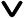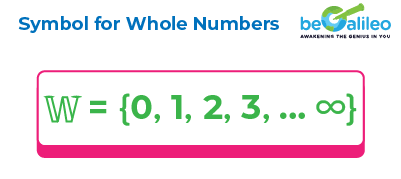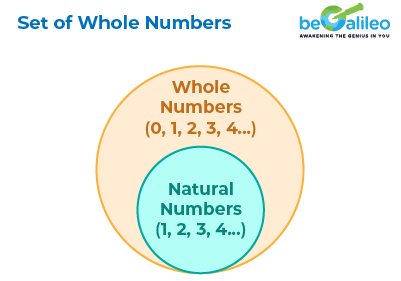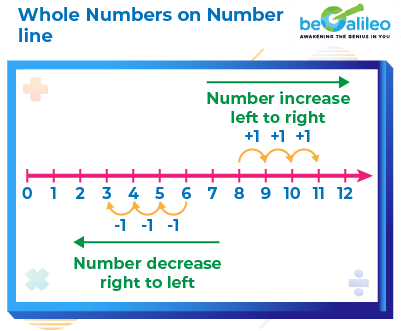>### Master Math with 1:1 Online Classes. Learn from Experts!# Whole Numbers• Definition of whole numbers
• Set of Whole Numbers
• Examples of Whole Numbers
• Real-World Examples of Whole Numbers
• Smallest and Largest Whole Number
• History of Whole Numbers
• Whole Numbers on a Number Line
• Properties of Whole Numbers
• Difference between Whole Numbers and Natural Numbers
• Solved Examples
• Practice Problems

The world of math started with counting numbers or natural numbers 1,2,3,4.. ..But soon there was a need to express quantity when there was nothing. For example if you had 5 apples and you ate them all, you are left with no apples. Math needs a way to represent this and hence came zero, ‘0’ and along with it the set of whole numbers.

## Definition of whole Numbers

Whole numbers is the collection of all natural numbers and 0. They do not include negative numbers, decimals or fractions. Whole numbers start from 0, 1, 2, 3..and continue till infinity.Whole numbers are defined as natural or counting numbers including zero.

### Set of Whole Numbers

The set of whole numbers is a collection of numbers that includes all the natural numbers, but it also includes zero.
In mathematical notation, the set of whole numbers is often represented asThis set starts from zero and includes all positive integers. It is read as 𝕎 is a set of all whole numbers from 0 to infinity.

{ } is the brackets used to denote the set.

∞ is the symbol used to represent infinity.

Note: Set of Natural Numbers is a subset of Set of Whole Numbers.### Examples of Whole numbers

Here are some examples of whole numbers:

0, 34, 5678, 870.

These numbers represent counts of objects or positions in a sequence.

## Real-World Examples of Whole Numbers

Counting : Whole numbers are used for counting objects, people, or anything that can be counted. For example, counting the number of students in a class or the apples in a basket.

Measurement : When measuring objects, whole numbers are often used. For example, the number of meters in a length or the number of liters in a container.

Budgeting and Finance : Whole numbers are important in financial calculations, such as calculating expenses, incomes, and budgets.

Sports and Games : Scores, rankings, and player numbers in sports are represented using whole numbers.

Coding and Computing : In computer programming, whole numbers are used for various purposes, including counting iterations and indexing elements.

## Smallest and Largest Whole Number

Smallest Whole number: The smallest whole number is 0. It's the starting number of the whole number system and represents the absence of quantity. Although it has no value, zero is used as a placeholder. Therefore, zero is a number that can neither be positive nor negative.

Largest Whole number: There is no definitive "largest" whole number. As the whole number goes infinitely, there is no greatest number. No matter how large a whole number may be, one can always add 1 to it to obtain a new larger whole number. This provides a way to understand the availability of limitless whole numbers. This concept is known as the principle of infinity.## History of Whole Numbers

Whole numbers, as a concept, were not "discovered" by a single individual, but rather developed over time by various civilizations and mathematicians. The idea of counting and using whole numbers is deeply implanted in human history.

Various ancient civilizations, such as the Sumerians, Egyptians, and Indus Valley people, developed basic numeral systems that included symbols for representing quantities. These systems often had symbols for the first few whole numbers.

The Indian mathematicians of ancient times, such as Aryabhata and Brahmagupta, made significant contributions to the development of the decimal numeral system, including the use of zero as a placeholder and the concept of positional notation.

Fibonacci, an Italian mathematician from the 13th century, introduced the Hindu-Arabic numeral system to Europe, which included the concept of whole numbers and zero. Mathematicians Pierre de Fermat, Carl Friedrich Gauss expanded the understanding of whole numbers' properties and relationships.

## Whole Numbers on a Number Line

Representing whole numbers on a number line is a visual way to understand the position and value of the numbers on a straight line. On the number line, the numbers are arranged in sequence at equal intervals along its length.The numbers increase as you move from left to right on the number line and decrease as you move backwards from right to left.## Properties of Whole Numbers

Properties of whole numbers play an important role in understanding the pattern of the whole numbers and establishing a relationship between the numbers and operations.
The four fundamental operations of whole numbers are addition, subtraction, multiplication, and division, giving rise to four main properties of whole numbers.

### Closure Property

When we add or multiply two whole numbers, we always get a whole number.So, the set of whole numbers is closed under addition and multiplication.

Example:
3 + 9 = 12 (a whole number)
3 \times 9 = 27 (a whole number)
3 + 0 = 3 (a whole number)
3 \times 0 = 0 (a whole number)

### Commutative Property

The result of addition or multiplication of whole numbers remains the same, regardless of the order in which the numbers are arranged.
So, the commutative property holds for addition and multiplication of whole numbers.

Example:
3 \times 9 =9 \times 3 = 12
3 + 9 = 9 + 3 = 12

### Associative Property

The sum and product of whole numbers remain constant, irrespective of how we arrange or group the numbers. The associative property can be conveniently illustrated using parentheses.

1. a. 4 + (3 + 2) = 4 + 5 = 9
b. (4 + 3) + 2 = 7 + 2 = 9
Thus, 4 + (3 + 2) = (4 + 3) + 2
2. a. 4 \times (3\times 2 )=4\times6 =24
b. (4 \times3) \times 2 =4\times6 =24
Thus, 4 \times(3 \times 2 )=(4\times3)\times2

Both addition and multiplication of whole numbers exhibit the associative property.

### Distributive Property

The distributive property in math lets us distribute or share the multiplication of a whole number over the addition or subtraction of other whole numbers inside parentheses. This handy rule simplifies equations and makes them easier to solve.

Example:
4\times(8+6)=4\times14=56 (a whole number)
4\times(8+6)=(4\times8)+(4\times6)=32+24=56 (a whole number)
Thus, 4\times(8+6)=(4\times8)+(4\times6)

The whole number 0 serves as the additive identity. Adding 0 to any whole number doesn't change its value.

Example:
8 + 0 = 0 + 8 = 8

For any whole number, there exists an additive inverse that, when added to the number, gives zero.
For a whole number ‘a’, the additive inverse is ‘ -a’.

Example:
8 + (-8) = 0

### Multiplicative Identity

The whole number 1 serves as the multiplicative identity. Multiplying any whole number by 1 doesn't change its value.

Example:
4\times1=4

## Difference between Whole Numbers and Natural Numbers

Whole numbers and natural numbers are both types of numbers in mathematics, but they have slight differences in their definitions and the set of numbers they include.

Natural Numbers Whole Numbers
Numbers starting from 1, 2, 3, 4, … ∞ are natural numbers. Numbers starting from 0, 1, 2, 3, … ∞ are whole numbers.
The set of natural numbers is: N = {1, 2, 3, 4, 5, ...} The set of whole numbers is: W = {0, 1, 2, 3, 4, ...}
1 is the least natural number 0 is the least whole number.
All whole numbers are considered as natural numbers except 0. All natural numbers are considered as whole numbers.
Example: length, time, weight, and so on Example: phone numbers, TV channels, speedometer, and so on

## Solved Examples

### Q1. Which of the following is a whole number?

1. a) -1
2. b) 0
3. c) \frac{1}{2}
4. d) 3.14

Solution: The correct answer is b) 0
Whole numbers are a set of numbers that include all the natural numbers along with zero.
Hence, 0 is the correct answer.

### Q2. Which of the following sets contains only whole numbers?

1. a) {-2, 0.5, 3, -5}
2. b) {1, 2, 3, 4}
3. c) {0.25, 0.5, 0.75, 1}
4. d) {-1, -2, -3, -4}

Solution: The correct answer is b) {1, 2, 3, 4}
Whole numbers are a set of numbers that include all the natural numbers along with zero.
Hence, The set {1, 2, 3, 4} is the correct answer.

### Q3. What is the result of multiplying any whole number by 0?

1. a) 1
2. b) The same whole number
3. c) 0
4. d) 10

Solution: The correct answer is c) 0
When you multiply any whole number by 0, the result will always be 0.

### Q4. Which of the following operations will always result in a whole number?

1. a) Addition of two whole numbers
2. b) Division of two whole numbers
3. c) Square root of a whole number
4. d) Multiplication of two fractions

When you add two whole numbers, the result will always be a whole number. Hence, the correct answer is addition of two whole numbers.

### Q5. Which of the following numbers is NOT a whole number?

1. a) 5
2. b) 0
3. c) 7
4. d) 1.5

Solution: The correct answer is d) 1.5
Whole numbers consist of all the natural numbers along with zero.
Hence, 1.5 is not a whole number.

## FAQs - Whole Numbers

Whole numbers are a set of numbers that includes all the positive integers (numbers without decimal or fractional parts) starting from zero and counting upward indefinitely (0, 1, 2, 3, ...).

No, negative numbers are not considered whole numbers. Whole numbers only include non-negative integers, starting from zero and counting upwards.

No, fractions and decimals are not considered whole numbers. Whole numbers are integers without any fractional or decimal parts.

Yes, zero is considered a whole number. It's the starting point for whole numbers, and they include all the positive integers as well as zero itself.

Natural numbers are a subset of whole numbers. Natural numbers start from 1 and include all positive integers (1, 2, 3, ...), while whole numbers start from 0 and include both zero and all positive integers (0, 1, 2, 3, ...).

Yes, whole numbers can be either odd or even. An odd number is one that is not divisible by 2 and leaves a remainder of 1 when divided by 2. An even number is divisible by 2 and leaves no remainder when divided by 2.

Integers include all positive and negative whole numbers along with zero. Whole numbers only include non-negative integers (zero and positive integers), while integers include both positive and negative numbers as well.

Prime whole numbers are whole numbers greater than 1 that have no divisors other than 1 and themselves. Examples include 2, 3, 5, 7, 11, 13, and so on.

Yes, whole numbers can be represented on a number line. Each whole number is represented by a point on the line, and the distance between consecutive whole numbers is the same.

Whole numbers have several properties, including closure under addition and multiplication, associativity, commutativity, the existence of an identity element (0 for addition, 1 for multiplication), and the distributive property of multiplication over addition.Whole numbers are used for counting objects, people, or anything that can be counted. For example, counting the number of students in a class or the apples in a basket.